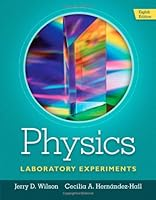# Physics Laboratory Experiments, 8th Edition## Book Description

This market-leading manual for the first-year physics laboratory course offers a wide range of class-tested experiments designed specifically for use in small to mid-size lab programs. A series of integrated experiments emphasizes the use of computerized instrumentation and includes a set of "computer-assisted experiments" that allow you to gain experience with modern equipment. By analyzing data through two different methods, learners gain a greater understanding of the concepts behind the experiments. The Eighth Edition is updated with four new economical labs and thirty new Pre-Lab Demonstrations, designed to capture interest prior to the lab and requiring only widely available materials and items.

Ch 1: The Method and Thought
Ch 2: Experimental Uncertainty (Error) and Data
Ch 3: Measurement Instruments (Mass, Volume, and Density)
Ch 4: (GL) Simple Pendulum Parameters (Angle, Mass, Length, and Damping)
Ch 5: (TI-GL/CI) Uniformly Accelerated Motion: Measurement of g
Ch 6: The Addition and Resolution of Vectors: The Force Table
Ch 7: (TI-GL/CI) Newton's Second : The Atwood Machine
Ch 8: (TI/CI) Conservation of Linear Momentum
Ch 9: (GL) Projectile Motion: The Ballistic Pendulum
Ch 10: Centripetal Force
Ch 11: (TI/CI) Friction
Ch 12: (GL) Work and Energy
Ch 13: Potential Energy of a
Ch 14: (GL) Torques, Equilibrium, and Center of Gravity
Ch 15: (GL) Simple Machines: Mechanical Advantage
Ch 16: (TI/CI) Simple Harmonic Motion
Ch 17: Standing Waves in a String
Ch 18: Temperature and Thermometer Calibration
Ch 19: The Thermal Coefficient of Linear Expansion
Ch 20: Specific Heats of Metals
Ch 21: Archimedes' Principle: Buoyancy and Density
Ch 22: Fields and Equipotentials
Ch 23: (TI/CI) Ohm's Law
Ch 24: The Measurement of Resistance: Ammeter-Voltmeter Methods and Wheatstone Bridge Method
Ch 25: The Temperature Dependence of Resistance
Ch 26: (TI/CI) Resistances in Series and Parallel
Ch 27: Joule Heat
Ch 28: The RC Time Constant (Manual Timing)
Ch 29: (TI/CI) The RC Time Constant ( Timing)
Ch 30: Reflection and Refraction
Ch 31: Spherical Mirrors and Lenses
Ch 32: (TI) Polarized Light (CI) Malus's Law
Ch 33: The Prism Spectrometer: Dispersion and the Index of Refraction
Ch 34: Line Spectra and the Rydberg Constant
Ch 35: (TI) The Transmission Diffraction Grating: Measuring the Wavelengths of Light (CI) Single-Slit and Double-Slit Diffraction
Ch 36: Detection of Nuclear Radiation: The Geiger Counter
Ch 38: The Absorption of Nuclear Radiation
Appendix A: Material Properties
Appendix B: Mathematical and Constants
Appendix C: Absolute Deviation and Mean Absolute Deviation
Appendix D: Standard Deviation and Method of Least Squares
Appendix E: Graphing Exponential Functions